HOMEPAGE for Eighth-Root of [ 1 ]

# Brief Notes on the Eighth-Root of [ 1 ]

### Key Findings and Introduction :

• During a brief study of { Complex Numbers } , the question arose :

• as to whether [ i ] and [ -i ] can be freely interchanged , without adverse effects .

• On further investigations , the Finiding here is simply this :

• If the { eighth-roots of [ 1 ] } are :

•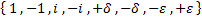then each of the following 3 sets , i.e. the sets :

•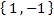,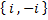,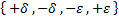;

would each exhibit a different and distinct { combinatorics-symmetry pattern } .

### The Investigation :

• We start-off our investigation with the { Fermat Little Theorem } :

•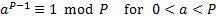where [ P ] is an { odd prime number } and [ a ] is a { positive integer } less than [ P ] .

We then bring-in an extension thereof previously discussed in { Approach to the Characteristics Of Numbers } , namely :

• For the { odd prime number } [ P ] , we set up the value [ Q ] such that :

•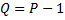and the equation :

•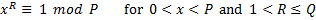has solutions , other than [ 1 ] , if and only if [ R ] is divisible into [ Q ] .

• For the { primer number } [ 233 ] , with { 233 = 8 x 29 + 1 } , the equation :

•has solutions other than [ 1 ] .

We then bring-in this { tree-diagram } on-the-left , below , to give the 8 { roots } :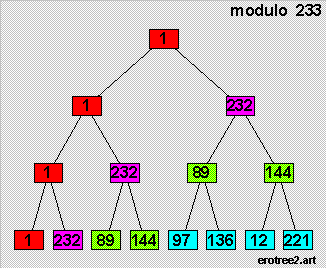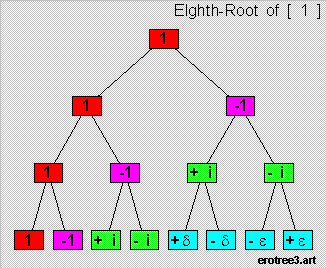• And the { tree-diagram } on-the-right , above , then gives the 8 { eighth-roots } of [ 1 ] , with :

•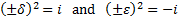• Let us now bring-in this equation for the diagram on-the-left :

•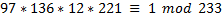and look at an equation of similar format , modelled therefrom , for the diagram on-the-right :

•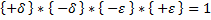We then have :

•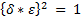yielding the relation :

•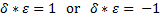• We shall now make a arbitrary choice here , namely , we set :

•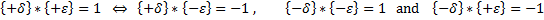### The { Counter-Multiplicative Conjugate } Relation :

• Let us now define , for the value [ w ] :

• the { Additive Conjugate } [ x ] , as the value satisfying the relation :

•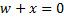• the { Multiplicative Conjugate } [ y ] , as the value satisfying the relation :

•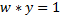• the { Counter-Multiplicative Conjugate } [ z ] , as the value satisfying the relation :

•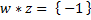We then bring-in this diagram for the 3 sets , i.e. the sets :

•,,;

whereby :

• each { horizontal pair } in the diagram below would depict a { additive conjugate } relation ,
• each { vertical pair } in the diagram below would depict a { muliplicative conjugate } relations .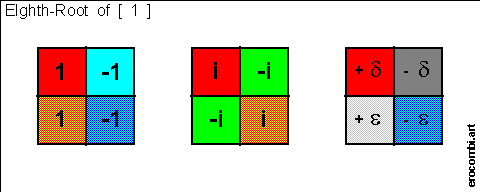• And each { cross-diagonal pair } here would depict a { counter-multiplicative conjugate } relation .

Let us now bring-in this set of 3 diagrams from { An Approach to the I-CHING } ,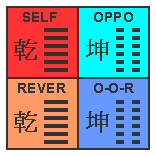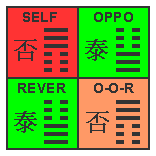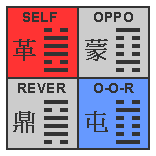CLEARLY , each of the 3 sets shows a different and distinct { combinatorics-symmetry pattern } .

### The { Tetrahedron Diagram } :

• Let us now put the four (4) elements in the set :

•at the 4 vertices of an { equilateral tetrahedron } , as per this diagram below :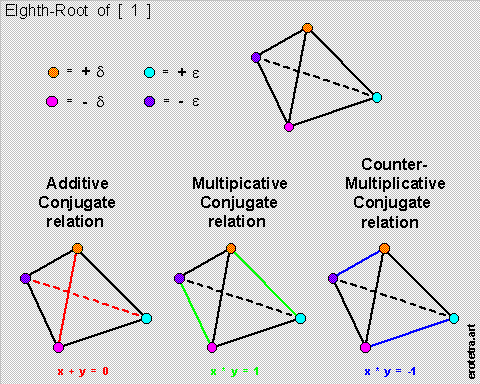We see here that each pair of opposite edges would then depict a different { conjugate relation } :

• And there are only three (3) such relations , namley :

• the { additive conjugate } relation ,
• the { multiplicative conjugate } relation , and
• the { counter-multiplicative conjugate } relation .

Seems likely that all three are always all-important .

### The { Green Cross } :

• We now bring-in this diagram , which shows the positions of the 4 elements in the set :

•in the { imaginary plane } , on the right-hand-side .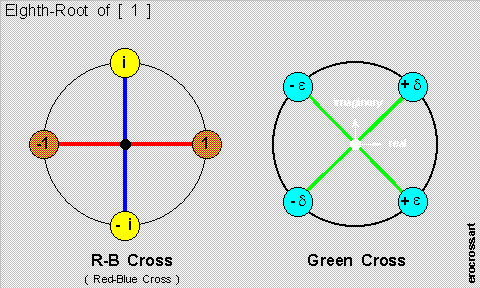• The question then arises as to whether :

• using the { R-B Cross } on-the-left above as the basis for { complex analysis }

is always superior to using the { Green Cross } on-the-right ?

May be not . And we shall leave this for the reader's further evaluation .

#### go to Top Of Page

• Riemann Zeta Function anyone ?

• Surely you must be joking .